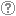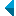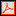# Iskanje po repozitorijuIskalni niz: išči po NaslovAvtorOpisKljučne besedePolno besediloLeto izida INALIIN NE išči po NaslovAvtorOpisKljučne besedePolno besediloLeto izida INALIIN NE išči po NaslovAvtorOpisKljučne besedePolno besediloLeto izida INALIIN NE išči po NaslovAvtorOpisKljučne besedePolno besediloLeto izida Vrsta gradiva: Vse vrste gradiv Habilitacijsko delo (m4) Višješolska diplomska naloga (m6) Specialistično delo (m3) Diplomsko delo * (dip) Magistrsko delo * (mag) Doktorska disertacija * (dok) Raziskovalni podatki ali korpus (data) * po starem in bolonjskem študiju Jezik: Vsi jezikiSlovenski jezikAngleški jezikNemški jezikHrvaški jezikSrbski jezikAfrikanski jezikAlbanski jezikArabski jezikArmenski jezikAzerbajdžanski jezikBolgarski jezikBosanski jezikČeški jezikČrnogorski jezikDanski jezikEstonski jezikFinski jezikFrancoski jezikGrški jezikGruzinski jezikIrski jezikItalijanski jezikJaponski jezikKatalonski jezikKazaški jezikKitajski jezikKorejski jezikLatinski jezikLatvijski jezikLitvanski jezikMadžarski jezikMakedonski jezikMalteški jezikNemški jezik (Avstrija)Nizozemski jezikNorveški jezikPoljski jezikPortugalski jezikRomunski jezikRuski jezikSlovanski jezik (ostali)Slovaški jezikSrbski jezik (cirilica)Španski jezikŠvedski jezikTurški jezikUkrajinski jezikNi določenVečjezičniNeznan jezikOstali Išči po: RUP    FAMNIT - Fakulteta za matematiko, naravoslovje in informacijske tehnologije    FHŠ - Fakulteta za humanistične študije    FM - Fakulteta za management    FTŠ Turistica - Fakulteta za turistične študije - Turistica    FVZ - Fakulteta za vede o zdravju    IAM - Inštitut Andrej Marušič    PEF - Pedagoška fakulteta    UPR - Univerza na Primorskem    ZUP - Založba Univerze na PrimorskemCOBISS    Fakulteta za humanistične študije, Koper    Fakulteta za management Koper in Pedagoška fakulteta Koper    Fakulteta za vede o zdravju, Izola    Knjižnica za tehniko, medicino in naravoslovje, Koper    Turistica, Portorož    Znanstveno-raziskovalno središče Koper Opcije: Prikaži samo zadetke s polnim besedilom Ponastavi

 11 - 20 / 4441234567891011.Graph characterization of fully indecomposable nonconvertible (0, 1)-matrices with minimal number of onesMikhail Budrevich, Gregor Dolinar, Aleksandr Èmilevič Guterman, Bojan Kuzma, 2019, izvirni znanstveni članekKljučne besede: permanent, indecomposable matrices, graphsObjavljeno v RUP: 03.01.2022; Ogledov: 547; Prenosov: 28Polno besedilo (278,69 KB) 12.Total positivity of Toeplitz matrices of recursive hypersequencesTomislav Došlić, Ivica Martinjak, Riste Škrekovski, 2019, izvirni znanstveni članekKljučne besede: total positivity, totally positive matrix, Toeplitz matrix, Hankel matrix, hyperfibonacci sequence, log-concavityObjavljeno v RUP: 03.01.2022; Ogledov: 481; Prenosov: 24Polno besedilo (254,44 KB) 13.Regular polygonal systemsJurij Kovič, 2019, izvirni znanstveni članekKljučne besede: regular polygonal system, boundary code, face vector, symmetry group, reconstructibility from the boundaryObjavljeno v RUP: 03.01.2022; Ogledov: 496; Prenosov: 17Polno besedilo (353,82 KB) 14.Splittable and unsplittable graphs and configurationsNino Bašić, Jan Grošelj, Branko Grünbaum, Tomaž Pisanski, 2019, izvirni znanstveni članekOpis: We prove that there exist infinitely many splittable and also infinitely many unsplittable cyclic ▫$(n_3)$▫ configurations. We also present a complete study of trivalent cyclic Haar graphs on at most 60 vertices with respect to splittability. Finally, we show that all cyclic flag-transitive configurations with the exception of the Fano plane and the Möbius-Kantor configuration are splittable.Ključne besede: configuration of points and lines, unsplittable configuration, unsplittable graph, independent set, Levi graph, Grünbaum graph, splitting type, cyclic Haar graphObjavljeno v RUP: 03.01.2022; Ogledov: 498; Prenosov: 19Polno besedilo (355,79 KB) 15.Linking rings structures and semisymmetric graphs : combinatorial constructionsPrimož Potočnik, Steve Wilson, 2018, izvirni znanstveni članekKljučne besede: graphs, automorphism group, symmetry, locally arc-transitive graphs, symmetric graphs, cycle structure, linking ring structureObjavljeno v RUP: 03.01.2022; Ogledov: 519; Prenosov: 18Polno besedilo (397,55 KB) 16.Combinatorial configurations, quasiline arrangements, and systems of curves on surfacesJürgen Bokowski, Jurij Kovič, Tomaž Pisanski, Arjana Žitnik, 2018, izvirni znanstveni članekKljučne besede: pseudoline arrangement, quasiline arrangement, projective plane, incidence structure, combinatorial configuration, topological configuration, geometric configuration, sweep, wiring diagram, allowable sequence of permutations, maps on surfacesObjavljeno v RUP: 03.01.2022; Ogledov: 416; Prenosov: 17Polno besedilo (3,66 MB) 17.Semiregular automorphisms in vertex-transitive graphs with a solvable group of automorphismsDragan Marušič, 2017, izvirni znanstveni članekOpis: It has been conjectured that automorphism groups of vertex-transitive (di)graphs, and more generally 2-closures of transitive permutation groups, must necessarily possess a fixed-point-free element of prime order, and thus a non-identity element with all orbits of the same length, in other words, a semiregular element. The known affirmative answers for graphs with primitive and quasiprimitive groups of automorphisms suggest that solvable groups need to be considered if one is to hope for a complete solution of this conjecture. It is the purpose of this paper to present an overview of known results and suggest possible further lines of research towards a complete solution of the problem.Ključne besede: solvable group, semiregular automorphism, fixed-point-free automorphism, polycirculant conjectureObjavljeno v RUP: 03.01.2022; Ogledov: 539; Prenosov: 17Polno besedilo (235,26 KB) 18.A note on acyclic number of planar graphsMirko Petruševski, Riste Škrekovski, 2017, izvirni znanstveni članekOpis: The acyclic number ▫$a(G)$▫ of a graph ▫$G$▫ is the maximum order of an induced forest in ▫$G$▫. The purpose of this short paper is to propose a conjecture that ▫$a(G)\geq \left( 1-\frac{3}{2g}\right)n$▫ holds for every planar graph ▫$G$▫ of girth ▫$g$▫ and order ▫$n$▫, which captures three known conjectures on the topic. In support of this conjecture, we prove a weaker result that ▫$a(G)\geq \left( 1-\frac{3}{g} \right)n$▫ holds. In addition, we give a construction showing that the constant ▫$\frac{3}{2}$▫ from the conjecture cannot be decreased.Ključne besede: induced forest, acyclic number, planar graph, girthObjavljeno v RUP: 03.01.2022; Ogledov: 622; Prenosov: 15Polno besedilo (227,50 KB) 19.Classification of convex polyhedra by their rotational orbit Euler characteristicJurij Kovič, 2017, izvirni znanstveni članekOpis: Let ▫$\mathcal P$▫ be a polyhedron whose boundary consists of flat polygonal faces on some compact surface ▫$S(\mathcal P)$▫ (not necessarily homeomorphic to the sphere ▫$S^{2}$)▫. Let ▫$vo_{R}(\mathcal P), eo_{R}(\mathcal P)$▫, ▫$fo_{R}(\mathcal P)$▫ be the numbers of rotational orbits of vertices, edges and faces, respectively, determined by the group ▫$G = G_{R}(P)$▫ of all the rotations of the Euclidean space ▫$E^{3}$▫ preserving ▫$\mathcal P$▫. We define the ''rotational orbit Euler characteristic'' of ▫$\mathcal P$▫ as the number ▫$Eo_{R}(\mathcal P) = vo_{R}(\mathcal P) - eo_{R}(\mathcal P) + fo_{R}(\mathcal P)$▫. Using the Burnside lemma we obtain the lower and the upper bound for ▫$Eo_{R}(\mathcal P)$▫ in terms of the genus of the surface ▫$S(P)$▫. We prove that ▫$Eo_{R} \in \lbrace 2,1,0,-1\rbrace$▫ for any convex polyhedron ▫$\mathcal P$▫. In the non-convex case ▫$Eo_{R}$▫ may be arbitrarily large or small.Ključne besede: polyhedron, rotational orbit, Euler characteristicObjavljeno v RUP: 03.01.2022; Ogledov: 468; Prenosov: 16Polno besedilo (272,96 KB) 20.Vertex-transitive graphs and their arc-typesMarston D. E. Conder, Tomaž Pisanski, Arjana Žitnik, 2017, izvirni znanstveni članekOpis: Let ▫$X$▫ be a finite vertex-transitive graph of valency ▫$d$▫, and let ▫$A$▫ be the full automorphism group of ▫$X$▫. Then the arc-type of ▫$X$▫ is defined in terms of the sizes of the orbits of the stabiliser ▫$A_v$▫ of a given vertex ▫$v$▫ on the set of arcs incident with ▫$v$▫. Such an orbit is said to be self-paired if it is contained in an orbit ▫$\Delta$▫ of ▫$A$▫ on the set of all arcs of v$X$▫ such that v$\Delta$▫ is closed under arc-reversal. The arc-type of ▫$X$▫ is then the partition of ▫$d$▫ as the sum ▫$n_1 + n_2 + \dots + n_t + (m_1 + m_1) + (m_2 + m_2) + \dots + (m_s + m_s)$▫, where ▫$n_1, n_2, \dots, n_t$▫ are the sizes of the self-paired orbits, and ▫$m_1,m_1, m_2,m_2, \dots, m_s,m_s$▫ are the sizes of the non-self-paired orbits, in descending order. In this paper, we find the arc-types of several families of graphs. Also we show that the arc-type of a Cartesian product of two "relatively prime" graphs is the natural sum of their arc-types. Then using these observations, we show that with the exception of ▫$1+1$▫ and ▫$(1+1)$▫, every partition as defined above is \emph{realisable}, in the sense that there exists at least one vertex-transitive graph with the given partition as its arc-type.Ključne besede: symmetry type, vertex-transitive graph, arc-transitive graph, Cayley graph, cartesian product, covering graphObjavljeno v RUP: 03.01.2022; Ogledov: 495; Prenosov: 16Polno besedilo (475,17 KB)
Iskanje izvedeno v 0.14 sek.
Na vrh